+
Representing Skewed Data on a Graph

# Representing Skewed Data on a Graph

Author: Sophia Tutorial
##### Description:

Identify a graph for a skewed distribution.

(more)

Sophia’s self-paced online courses are a great way to save time and money as you earn credits eligible for transfer to many different colleges and universities.*

No credit card required

37 Sophia partners guarantee credit transfer.

299 Institutions have accepted or given pre-approval for credit transfer.

* The American Council on Education's College Credit Recommendation Service (ACE Credit®) has evaluated and recommended college credit for 33 of Sophia’s online courses. Many different colleges and universities consider ACE CREDIT recommendations in determining the applicability to their course and degree programs.

Tutorial
what's covered
This lesson discusses representing how skewed data is distributed. By the end of the lesson, you should be able to identify the mean, median, and mode on a skewed distribution. This lesson covers:
1. Skewed Distribution
2. Right Skewed Distribution
3. Left Skewed Distribution
4. Telling The Difference

## 1. Skewed Distribution

If you recall from a previous lesson, normal distributions have density curves that are symmetric and bell shaped. The mean, median, and mode of the normal distribution are all the same and equal to the center value of the density curve. However, there are situations where, unlike normal distributions, a distribution may not be symmetrical. We call these skewed distributions.

The first distribution that you see below is skewed. It reflects a situation in which there would be many values concentrated toward the lower end of the distribution relative to the higher end. This is typically how the housing market is distributed.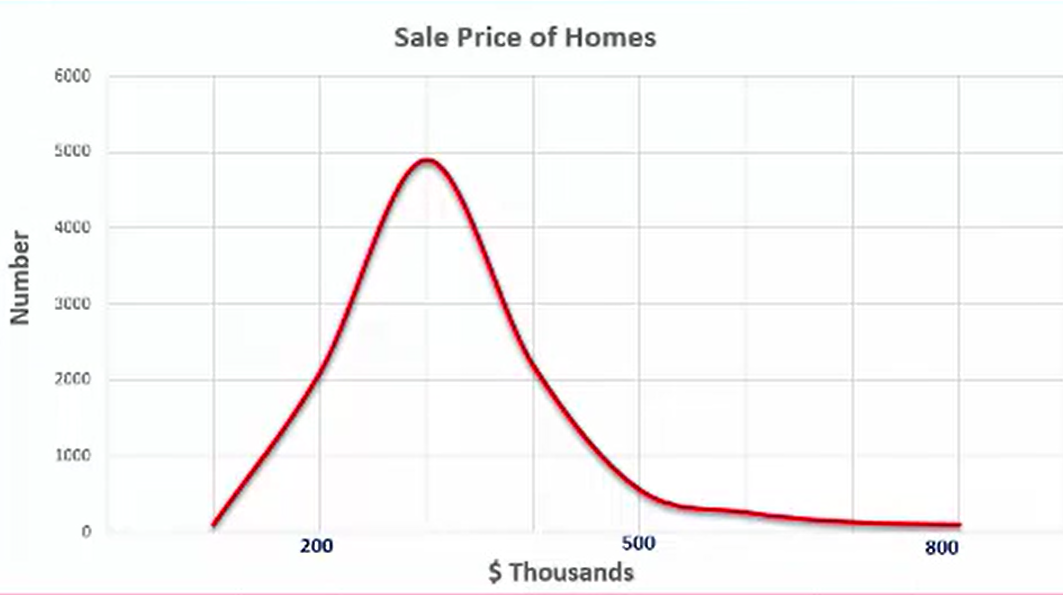This second distribution is skewed, and it reflects a situation in which there will be many values concentrated toward the higher end of the distribution relative to the lower end. A good example of this distribution would be the mileage on the odometers of used cars.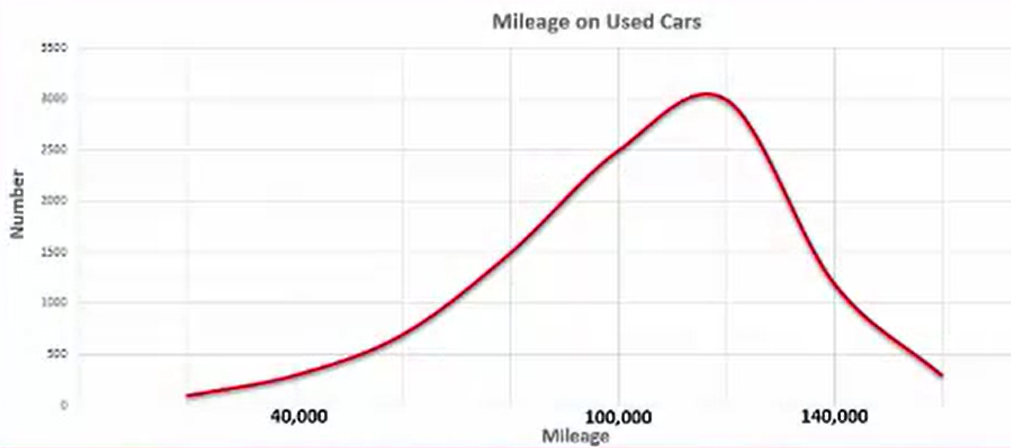## 2. Right Skewed Distribution

Refer back to the home values graph. On the bottom (horizontal) axis, you have the price listed in thousands of dollars. The range is from around 100,000 up to almost 800,000. The majority of the values are concentrated right around the \$300,000 mark. This is going to be a right skewed, or positively skewed, distribution curve.

Why is it right skewed? Because so many observations are around that \$300,000 point, but there are also a lot of homes priced much higher. That pulls the mean up while leaving the median or the middle value relatively low.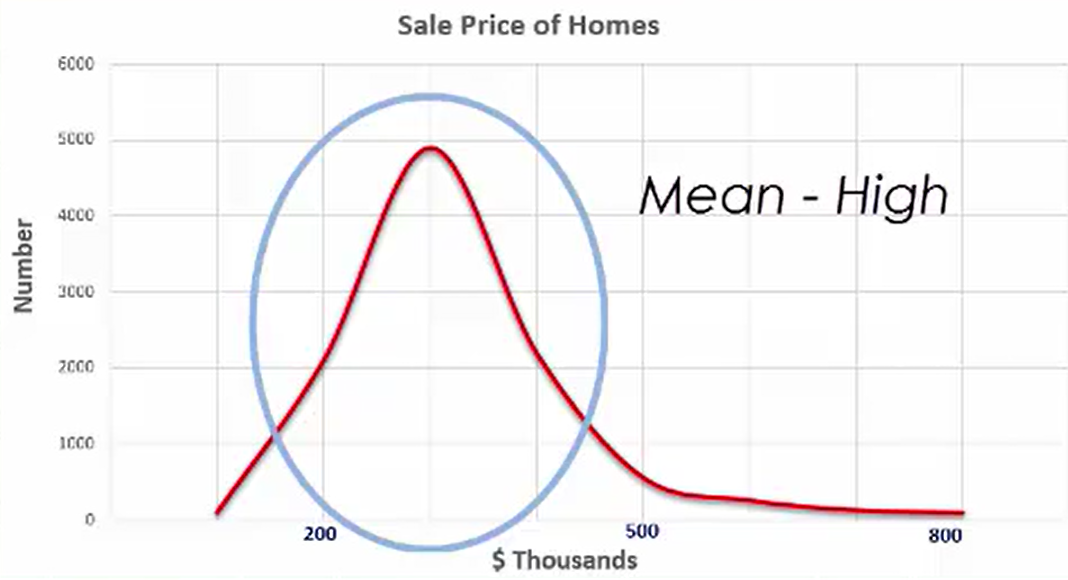In this case, the mode would be the smallest value, then the median, and then the mean. You’d read the graph along the horizontal axis from left to right.

When you look at a right skewed distribution, the far right-hand side is pointing to the right. Imagine it’s an arrow, with the tail indicating the direction of the skew.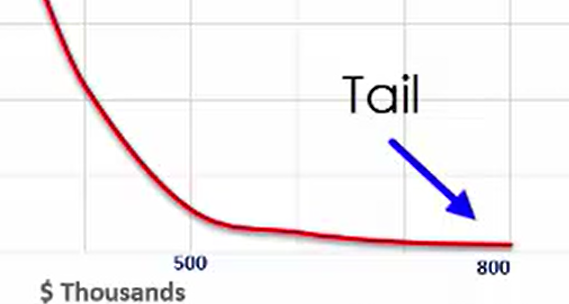The mean is \$400,000, which is higher than either the median (\$325,000) or the mode (\$300,000). This is a key indicator of a right , or a positively, skewed distribution.

Mode < Median < Mean

## 3. Left Skewed Distribution

A left skewed distribution, on the other hand, would be an example such as the mileage on used cars. If you look at the distribution of the mileage of used cars shown below, you notice that there are similarities to the previous graph. However, it’s not going to necessarily look exactly the same. This type of distribution is called a left skewed distribution, or a negatively skewed distribution.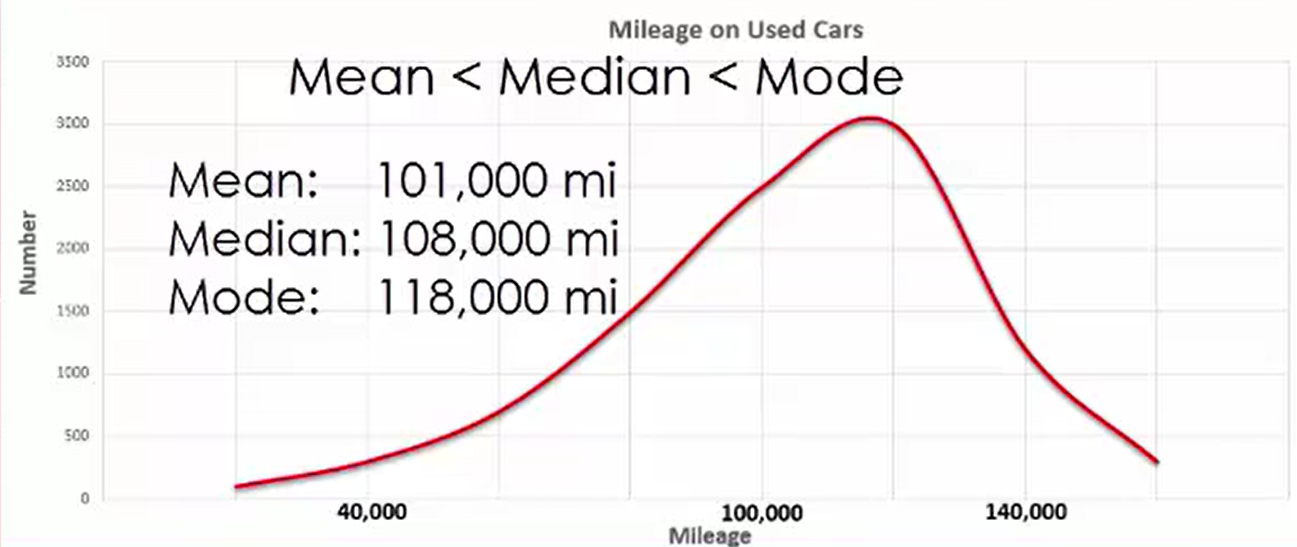In this case, the mode is greater than the median, and the median is greater than the mean.

You see a relatively low number on the lower end in terms of the value of miles on a car. This is because people might wait a lot longer to trade in their vehicles, which might mean they have higher mileage. You’d see a left skewed distribution. Notice that the tail points to the left.

## 4. Telling The Difference

So how do we identify differences between distributions? Look at this graph, which shows a normal distribution. It’s symmetrical. The mean, median, and mode are identical, and they’d be the value right there in the middle.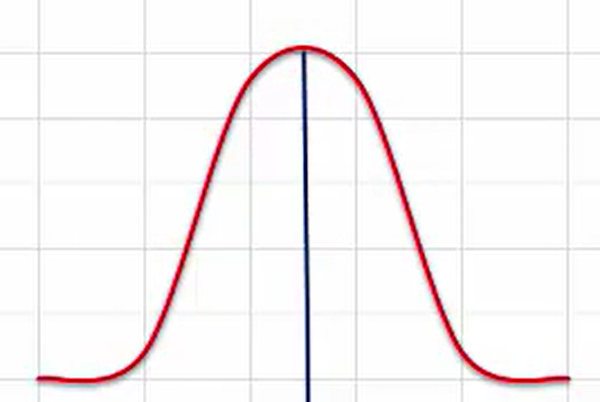Bank account balances are an example of skewed distribution, and they are right skewed. Some people have quite a bit of money in their bank account, but many don’t. In this situation, the mode and the median are going to be relatively low, and the mean’s going to be higher. That’s simply because there are some large balances that pull up the mean.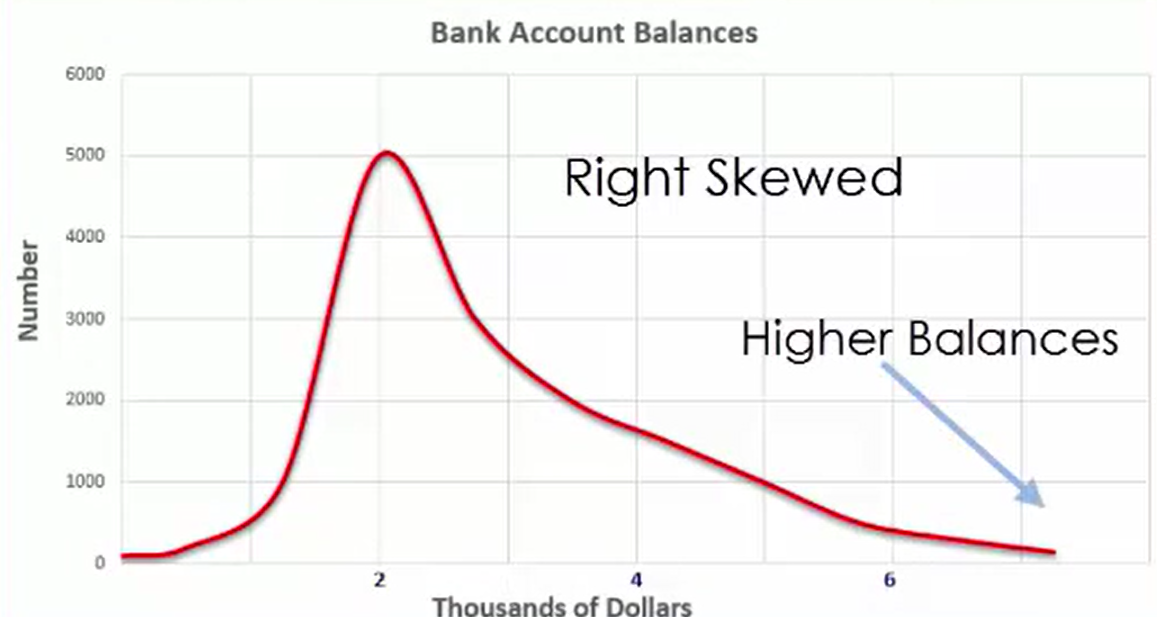For an example of a left skewed distribution, look at the heart rate of a sample of people. You’re going to see the heart rate measured in beats per minute, and the mean beats per minute is probably somewhere around the 70 or 80 mark. You will see some beats that are higher or lower than that. However, there will be relatively few people with a low heart rate. That’s going to bring you to a situation reflected by a left skewed distribution.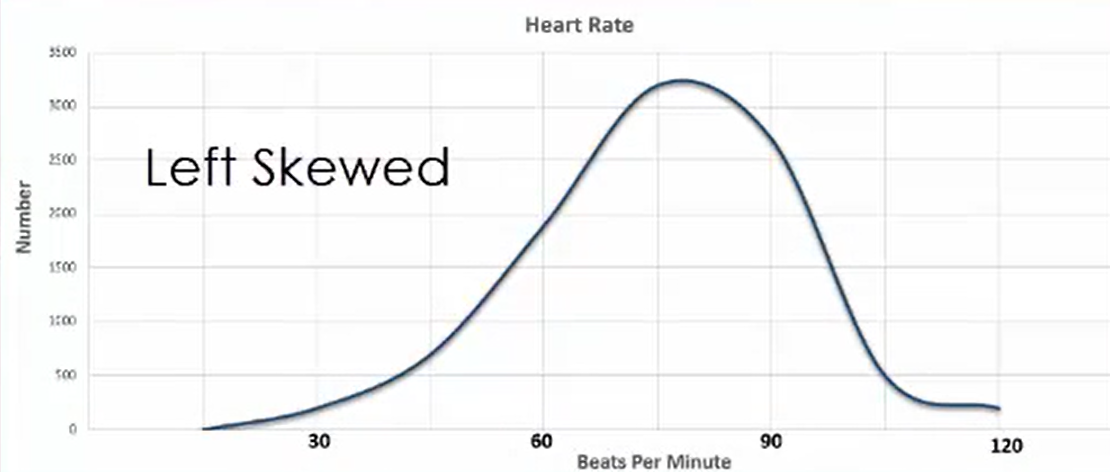How can you tell if a distribution is going to be skewed one way or the other?

It depends on the value of the mean, the median, and the mode.

If the mean is greater than the median and the mode, it is a right skewed distribution. If the mean is equal to the median and the mode, it’s a normal distribution. If the mean is less than the median and the mode, it is a left skewed distribution.

Situation Skewness
Mean > Median Right
Mean > Mode Right
Mean = Median Normal
Mean = Mode Normal
Mean < Median Left
Mean < Mode Left

summary
When distributions are not symmetrical, this is called a skewed distribution. Right skewed distribution occurs when the majority of observations are concentrated at lower values, but higher values bring up the mean. Left skewed distribution is the opposite. Telling the difference between the two requires you to look at the mean, median, and mode.

Source: This work is adapted from Sophia author Dan Laub.

Rating